Question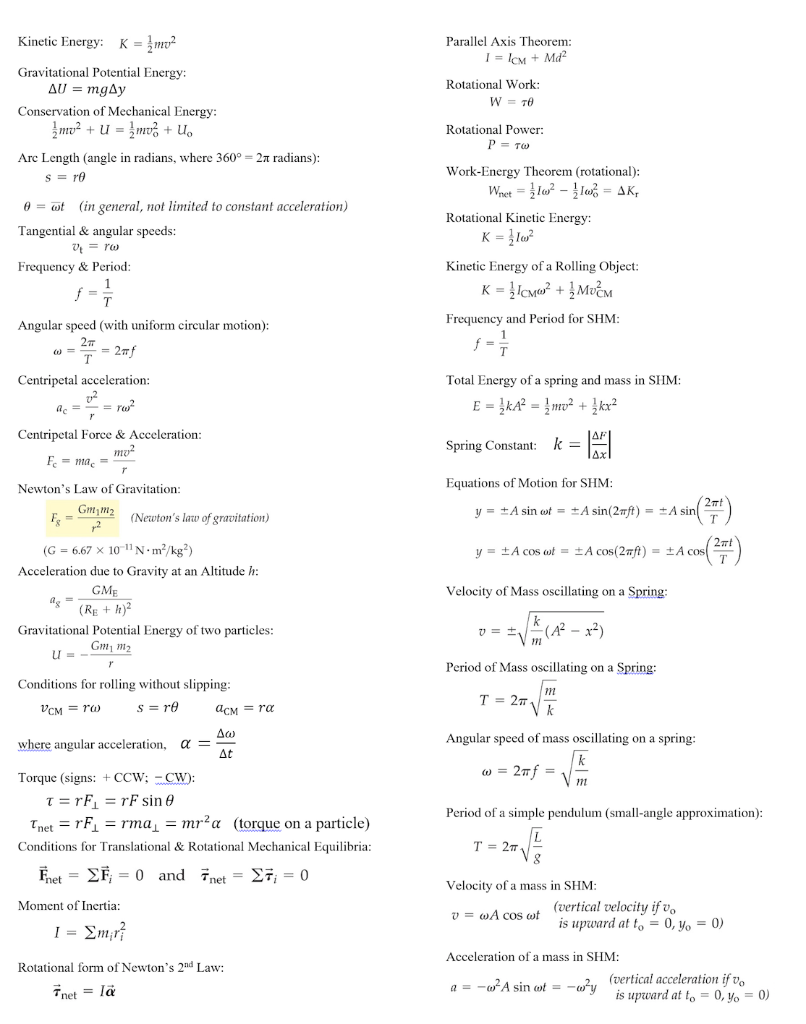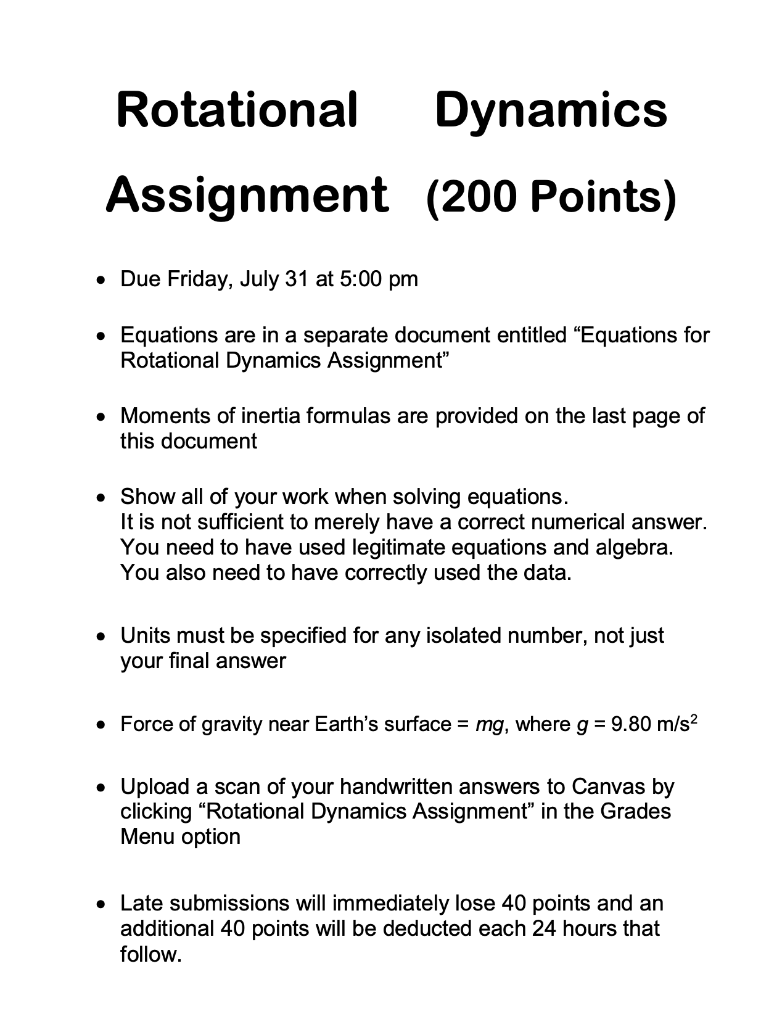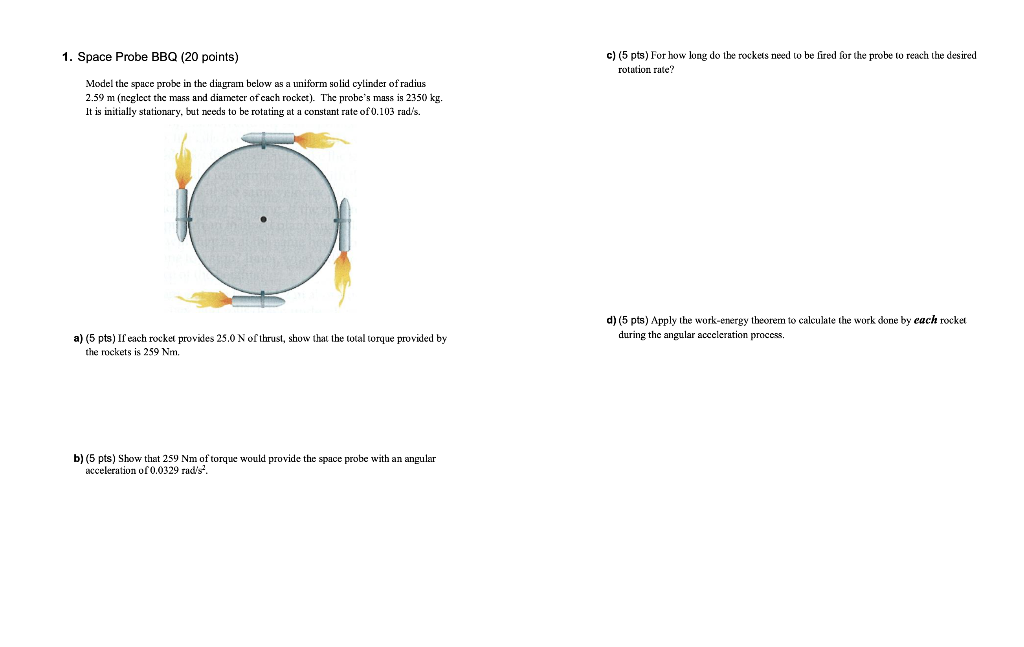Please see image and try to understand thanks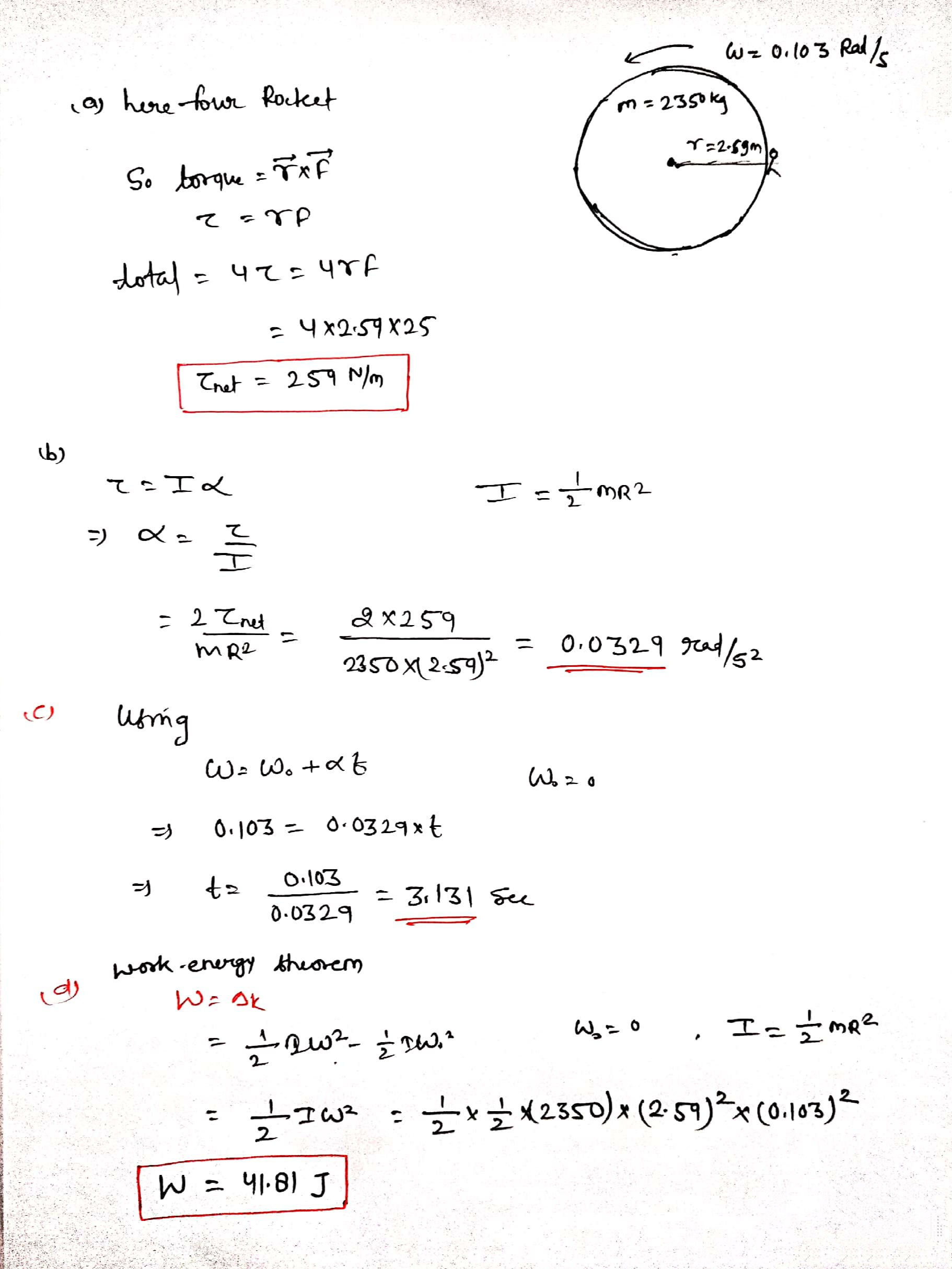#### Earn Coins

Coins can be redeemed for fabulous gifts.

Similar Homework Help Questions
• ### Please help answer all of question 6, thanks! Rotational Dynamics Assignment (200 Points) • Due Friday,...Please help answer all of question 6, thanks! Rotational Dynamics Assignment (200 Points) • Due Friday, July 31 at 5:00 pm Equations are in a separate document entitled “Equations for Rotational Dynamics Assignment” • Moments of inertia formulas are provided on the last page of this document • Show all of your work when solving equations. It is not sufficient to merely have a correct numerical answer. You need to have used legitimate equations and algebra. You also need to...

• ### Rotational Dynamics Assignment (200 Points) • Due Friday, July 31 at 5:00 pm Equations are in...Rotational Dynamics Assignment (200 Points) • Due Friday, July 31 at 5:00 pm Equations are in a separate document entitled “Equations for Rotational Dynamics Assignment” • Moments of inertia formulas are provided on the last page of this document • Show all of your work when solving equations. It is not sufficient to merely have a correct numerical answer. You need to have used legitimate equations and algebra. You also need to have correctly used the data. • Units must...

• ### • Equations are in a separate document entitled "Equations for Rotational Dynamics Assignment" • Moments of...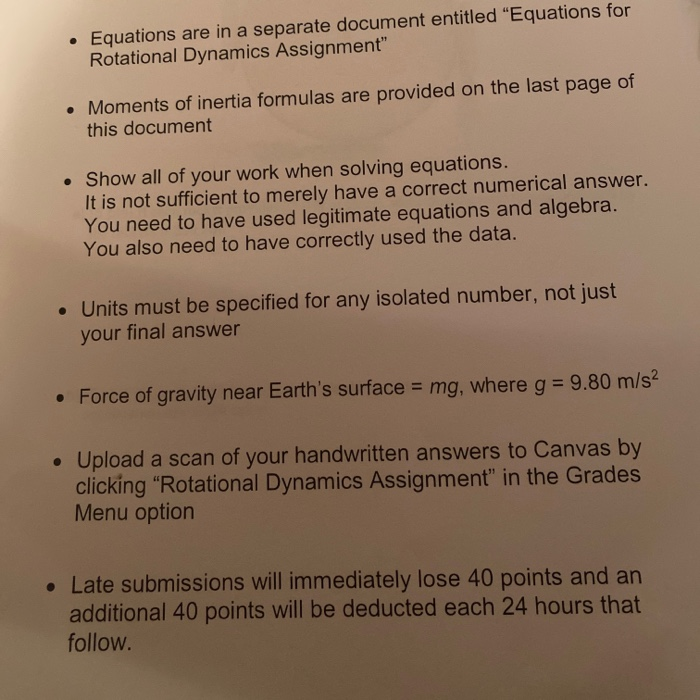• Equations are in a separate document entitled "Equations for Rotational Dynamics Assignment" • Moments of inertia formulas are provided on the last page of this document • Show all of your work when solving equations. It is not sufficient to merely have a correct numerical answer. You need to have used legitimate equations and algebra. You also need to have correctly used the data. • Units must be specified for any isolated number, not just your final answer •...

• ### Please show all work and ignore the work done SHM worksheet 2 To determine the angular...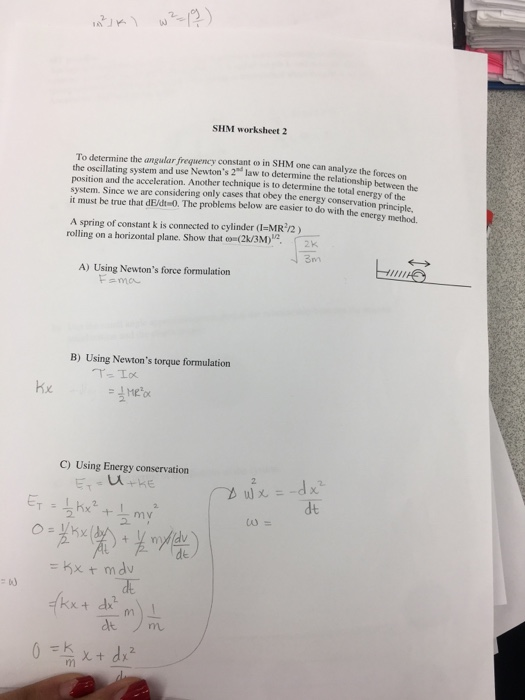Please show all work and ignore the work done SHM worksheet 2 To determine the angular frequency constant o in SHM one can analyze the forces on the oscillating system and use Newton's 2 law to determine the relationship hetwen position and the acceleration. Another technique is to determine the total system. Since we are considering only cases that obey the energy cons it must be true that dE/dt-0. The problems below are easier to do with the energy method...

• ### hectures Of 10.5 Cheder/ Homework: HW11: Parallel Axis Theorem And Torque & Rotational Dynamics Opcion Rotating...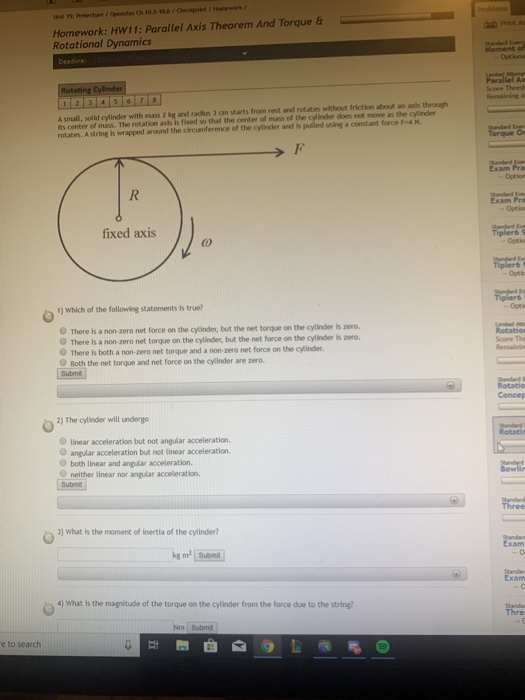hectures Of 10.5 Cheder/ Homework: HW11: Parallel Axis Theorem And Torque & Rotational Dynamics Opcion Rotating Cylinder Score Thred Asmall, solid cylinder with mass and radius om starts froen rest and rotates without friction about an als through its center of mass. The rotation and is feed so that the center of mass of the cylinder does not move as the cylinder rotates. A string is wrapped around the circunference of the cylinder and is pulled using a constant force...

• ### Homework: HW11: Parallel Axis Theorem And Torque Rotational Dynamics Limited Attempt Cluster Item Grade cluster Rotational...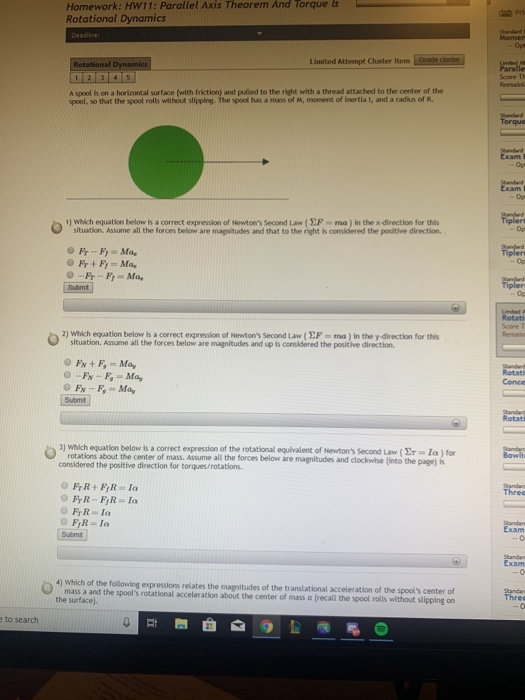Homework: HW11: Parallel Axis Theorem And Torque Rotational Dynamics Limited Attempt Cluster Item Grade cluster Rotational Dynamics Paralle Score Ti A spoolis on a horizontal surface with friction) and pulled to the night with a thread attached to the center of the spool, so that the spool rolls without slipping the spool has a mans of M, moment of inertial, and a radius of R. Torque cam Tipleri 1) Which equation below is a correct expression of Newton's Second Law...

• ### Please answer all of question 3 Rotational Dynamics Assignment (200 Points) • Due Friday, July 31...Please answer all of question 3 Rotational Dynamics Assignment (200 Points) • Due Friday, July 31 at 5:00 pm Equations are in a separate document entitled “Equations for Rotational Dynamics Assignment” • Moments of inertia formulas are provided on the last page of this document • Show all of your work when solving equations. It is not sufficient to merely have a correct numerical answer. You need to have used legitimate equations and algebra. You also need to have correctly...

• ### 1) The parallel axis theorem provides a useful way to calculate the moment of inertia I...

1) The parallel axis theorem provides a useful way to calculate the moment of inertia I about an arbitrary axis. The theorem states that I = Icm + Mh2, where Icm is the moment of inertia of the object relative to an axis that passes through the center of mass and is parallel to the axis of interest, M is the total mass of the object, and h is the perpendicular distance between the two axes. Use this theorem and...

• ### Prelab 1: Consider the following system consisting of a falling mass m attached by a thread...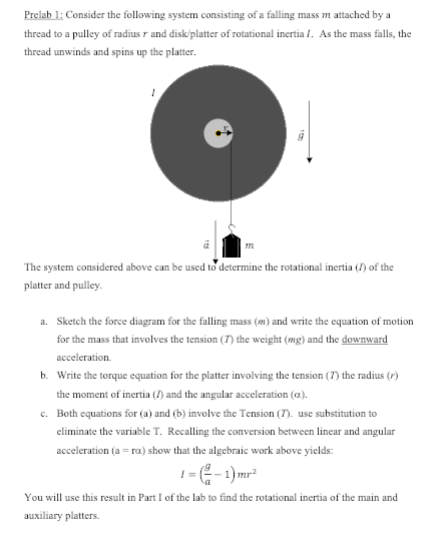Prelab 1: Consider the following system consisting of a falling mass m attached by a thread to a pulley of radius r and disk/platter of rotational inertiaI. As the mass falls, the thread unwinds and spins up the platter 17 The system considered above can be used to determine the rotational inertia () of the platter and pulley Sketch the force diagram for the falling mass (m) and write the equation of motion for the mass that involves the tension...

• ### solve using kinetic energy equations. ( Theorem of change in kinetic enegery) KE20. Figure shows a mechanism. Body 5...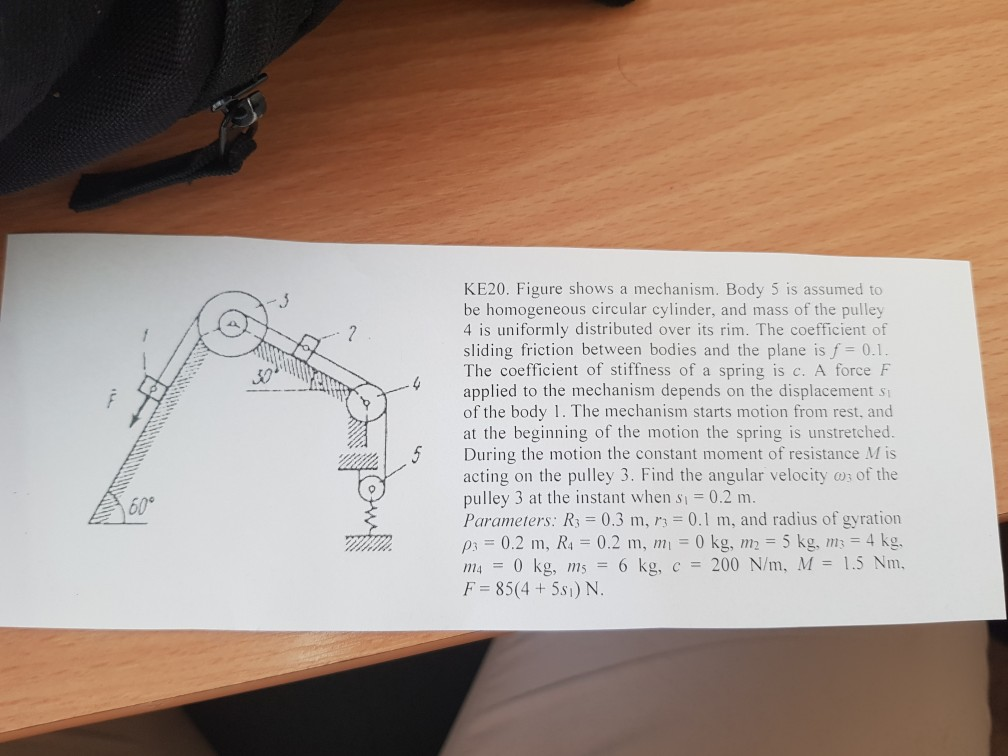solve using kinetic energy equations. ( Theorem of change in kinetic enegery) KE20. Figure shows a mechanism. Body 5 is assumed to be homogeneous circular cylinder, and mass of the pulley 4 is uniformly distributed over its rim. The coefficient of sliding friction between bodies and the plane is f 0.1 The coefficient of stiffness of a spring is c. A force F applied to the mechanism depends on the displacement s of the body 1. The mechanism starts motion...